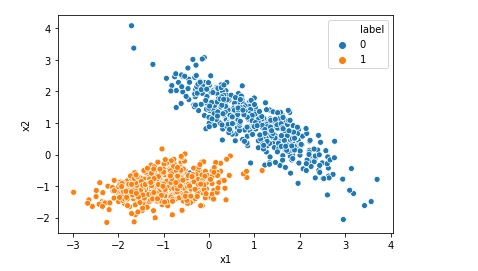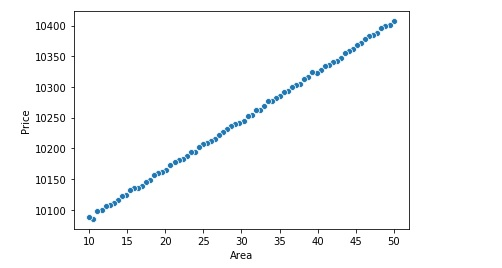# Linear Regression Synthetic Data using Make Regression

Though we have many datasets available on internet for implementing Linear Regression , many a times we may require to create a our own synthetic data. Scikit-Learn has a class called make regression , we can use this class to generate synthetic data for linear regression. from sklearn.datasets import make_regression # generate regression dataset x, … Continue reading Linear Regression Synthetic Data using Make Regression# What is R Squared for Linear Regression

For Linear Regression, R-squared is a statistical term which indicates how close the data are to the fitted regression line. R-Squared is also known as coefficient of determination. R-squared = Explained variation in data / Total variation in data R-squared = 1 - (RSS/TSS) RSS = Sum of squares of difference between predicted value and … Continue reading What is R Squared for Linear Regression# Implementing Logistic Regression in 10 lines in Python

Logistic Regression is one of the most popular Machine Learning algorithm used for the classification problems. It should be noted that though there is a regression word in the name of the algorithm Logistic Regression, it is used for classification problems. A use case of Logistic regression could be, based on the symptoms for a disease that a patient has Logistic … Continue reading Implementing Logistic Regression in 10 lines in Python

# How to Enable Intellisense or Autocomplete in Jupyter Notebook

No matter how good you are in programming with respect to a language like python you may not be able to remember all the functions names or syntax or function parameters. So you may require to use intellisense or autocomplete feature of Jupyter notebook while programming in pandas, python and similar libraries. In the below … Continue reading How to Enable Intellisense or Autocomplete in Jupyter Notebook

# How to Disable Warnings in Python and Pandas

Many a times when you run Python code in pandas you get warnings like below Disable or filter or suppress warning in python pandas However for various reasons you may want to disable or filter these warnings. For that use the below code import warnings warnings.filterwarnings("ignore") This will disable all the warnings and code will … Continue reading How to Disable Warnings in Python and Pandas# Linear Regression using sklearn in 10 lines

Linear regression is one of the most popular and fundamental machine learning algorithm. If relationship between two variables are linear we can use Linear regression to predict one variable given that other is known. For example if we are researching how the price of the house will vary if we change the area of the … Continue reading Linear Regression using sklearn in 10 lines